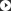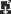VideoDownloads

## KESD

Main Market : North America South America Western Europe
Eastern Europe Eastern Asia Southeast Asia Middle East
Africa Worldwide
Brands : KESD
No. of Employees : 200~300
Annual Sales : 3000000-5000000
Year Established : 2003
Export p.c : 50% - 60%

## Industry News

You are here: » » » What is static?

# What is static?

Views:0     Author:Site Editor     Publish Time: 2017-09-07      Origin:Site

How does static happen

Static electricity is generated when two objects made of different electrically neutral materials touch and then separate.

1) Contact between objects A and B

Electrically neutral objects A and B (with equal amounts of positive and negative charges)

2) Fee transfer

When metals contact each other, electrons move from electrons with a small work function to electrons with a large work function. In other words, metals with smaller work functions will be positively charged, while metals with larger work functions will be negatively charged.

3) Separation between objects A and B

Subject A is negatively charged and Subject B is positively charged.Figure 1. Static electricity due to contact charging

When two objects touch and then separate, a static is generated. The combination of these two objects can be solid and solid, solid and liquid or liquid and liquid. Figure 2 shows an example.Figure 2. Static generation example

All the above phenomena involve contact and separation between two objects.Figure 3. Example of charging

The friction between two objects in a triboelectric sequence causes objects in the higher position in the sequence to be positively charged, while objects in the lower position are negatively charged. The friction between objects that are close together (B) and objects that are close together (A) are far away from each other (C) also generates a lot of static electricity. It should also be noted that the triboelectric series includes conductors, and the friction between the conductors also generates static electricity during insulation. As a result, the conductor will be charged.

While charging

When an object is electrostatically charged, an electric field is generated around the charged object, as shown in Figure 4.Figure 4. Electric field generated around a charged object

The electric field is the space in which the charge induces its force (Coulomb force) to work. Electric fields that do not change with time or space are called electrostatic fields. The number of power lines per unit area (density of lines) passing through a certain point indicates the strength of the electric field, and the tangent direction of the power line at that point indicates the direction of the electric field. The power line also has the following characteristics.

2. Lines do not intersect

3. No branch

4. Electric field is strong when power lines are dense

5. The electric field is uniform when the power lines are parallel

6. Vertically coming out from the conductor surface (entering)

Fig. 5 shows an example of a power line formed between two charged objects.(A) Power lines between objects with different polarities(B) Power lines between objects with the same polarity

Figure 5. Power line formed between two charged objects

Force lines are virtual lines used to visualize electric fields. Their use shows the state of the electric field formed between two charged objects, so it is easy to describe a static state, which is actually invisible. For example, Figure 6 shows the power line between two objects with different amounts of charge. The figure shows a power line applicable to the case where the charge amount Q1 of the charged object A is smaller than the charge amount Q2 of the charged object B. Since the two charged objects have the same size, the diameter of the power line per unit area of ​​the number of charged objects A is smaller than the line of the line per unit area of ​​the charged object B, and the electric field E2 is stronger than E1.

E1: Electric field strength on the conductor surfacePower line density: low à weak electric field

Charged conductive ball A

E2: Electric field strength on the conductor surfacePower line density: high à strong electric field

Charged conductive ball B

Figure 6. Power lines from two charged conductors with different amounts of charge

What is electrostatic force (Coulomb force)?

Static force (Coulomb force) is the source of static electricity. As shown in FIG. 7, the direction of the force between two charged objects differs depending on whether the objects have different polarities or the same polarity. The electrostatic force is attractive (a) when the polarities are different, and repulsive (b) when the two objects have the same polarity.(A) Force between objects with different polarities(B) Force between objects with the same polarity

Figure 7. Electrostatic force between two charged objects

As shown in formula (1), the strength of the electrostatic force (Coulomb force) is proportional to the product of the charge amounts of two charged objects, and is inversely proportional to the square of the distance between the two objects.

FQ1 ·Q2 /r2 ·········· (1)

Static induction is also caused by electrostatic forcesFigure 8. Electrostatic induction due to electrostatic forces

Electrostatic discharge is also caused by electrostatic force (Coulomb force)Figure 9. The generation of electrostatic discharge caused by electrostatic force

The electric field around a charged object accelerates the negative ions (electrons) in it by electrostatic force (Coulomb force), which has kinetic energy (wv). When these negative ions strike gas molecules in the electric field, the following formula holds,

Ionization energy of gas molecules<负离子的动能（wv）（2）>

Electrons are sputtered out of gas molecules, thereby generating negative and positive ions. If the resulting negative ions are subjected to electrostatic forces, the gas molecules are ionized, and the negative ions are accelerated in the electric field with their kinetic energy satisfying the condition expressed by the above formula (2). Repeating this process results in electrostatic discharge.

Conductor's charge distribution is also affected by electrostatic force (Coulomb force)

1) The charge provided to the electrically neutral conductor is distributed on the surface of the conductor. In other words, the given charge does not exist inside the conductor.
Suppose that four negative charges are given to the conductor, making them negatively charged, as shown in Figure 10. If four charges are provided inside the conductor (a), the electrostatic force (repulsive force) will act in it, forcing them to be distributed on the conductor surface.2) As described in the previous section, the charge imparted to the electrically neutral conductor is distributed on the surface of the conductor. These charges are distributed in such a way that the surface will be equipotential. In other words, a given charge will not exist inside the conductor. The distribution of charges on the conductor should make the surface equipotential.If the conductor surface has a potential distribution (a), a potential gradient (electric field Ex) is generated along the surface. Ex moves the charge on the surface of the conductor along the surface to the part with a low potential, thereby increasing the potential of the part. This movement of charge continues until the surface becomes equipotential.
As a result, the electric field coming out (entering) from the conductor surface is perpendicular to the surface (b).

(B) Charges distributed in such a way that the surface of the conductor is equipotential(B) Charges distributed in such a way that the surface of the conductor is equipotential

Figure 11. Charges are distributed on the conductor surface in such a way that the surface is equipotential.

Examples of problems caused by static electricityInquire:Ionization ChinaKESD.COM